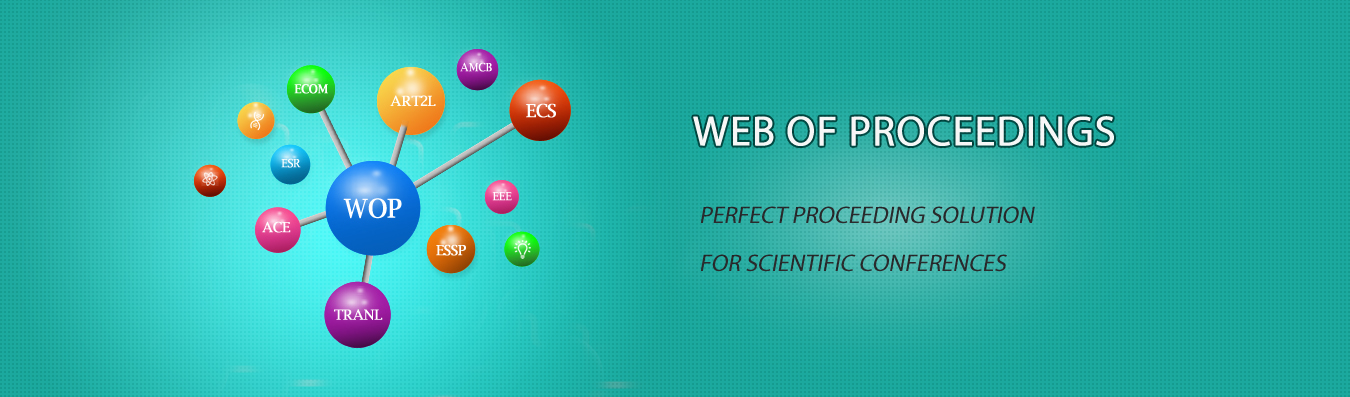The best way to conference proceedings by Francis Academic Press### Research on the Display of Matlab Mathematical Modeling to Higher Vocational College Students' Quality Improvement

DOI: 10.25236/ietrc.2020.147

#### Author(s)

Yuanzhen Huang, Xiaohui Liu

Xiaohui Liu

#### Abstract

In recent years, MATLAB mathematical modeling competition has been more and more popular among college students, and various MATLAB mathematical modeling activities have been carried out in various higher vocational colleges with MATLAB mathematical modeling competition as the carrier. MATLAB mathematical modeling is a link linking mathematics and life, and is also a bright spot in higher vocational mathematics education. Cultivating students' mathematical modeling ability of MATLAB can not only cultivate students' comprehensive ability, improve students' mathematical literacy, but also stimulate students' learning enthusiasm. Starting from the main functions and easy learning characteristics of MATLAB software, this paper expounds the importance of MATLAB software, and explains the necessity and possibility for higher vocational students to master MATLAB and the significance of learning this software well for mathematical modeling of higher vocational MATLAB.

#### Keywords

Matlab, Mathematical modeling, Higher vocational students, Quality improvement